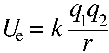Although molecules are quantum electrodynamic systems, classical electrostatics provides an extremely helpful 'rule of thumb' framework for interpreting potential energy changes at the molecular level. At heart, the discussion which follows involves comparative analysis of the molecular forms at hand in the light of the following basic formula for electrostatic potential energy:Let us develop an example to show how a basic understanding of electrostatics can help interpret a chemical equilibrium problem, let us take an example from acid-base chemistry. Why is acetic acid a stronger acid than ethanol, and why is trifluoroacetic acid a stronger acid than acetic acid? In other words, let us examine the strength of these three acids, from weakest to strongest: ethanol, acetic acid, and trifluoroacetic acid.

To begin, part of any examination of the strength of an acid is an investigation of the stability of the conjugate base. Typically, the ionization of an acids yields an anionic conjugate base. As a general rule, the lower the internal energy of this negatively charged product, generally, the more thermodynamically favored will be ionization, and therefore, the stronger the acid. In other words, something that causes the energy of the negatively charged conjugate base to be lower is going to tend to increase the acidity.

For example, increasing acidity in the progression from ethanol to acetic acid to fluoroacetic acid can be interpreted in terms of the decreased electrostatic potential energy of the negatively charged product. First, compare the conjugate base of ethanol (ethoxide) to the conjugate base of acetic acid (acetate). The big difference is that acetate is a resonant form, while ethoxide is not. Although the details are a bit more complicated, resonance allows the electron charge to spread out. With resonance stabilization, the acetate anion has lower energy vis-a-vis acetic acid than ethoxide does vis-a-vis ethanol. This lower electrostatic potential energy encourages spontaneous formation.

Moving from acetic acid to the even stronger acid, trifluoroacetic acid, we see further stabilization by induction in the trifluoroacetate anion. Induction enables opposite charges to draw closer together, the negative electrons being pulled inward by the powerful fluorine nucleus represents a different kind of decrease in electrostatic potential energy (electrostatic potential energy can decrease when like charges spread apart and when unlike charges move together).

In summary, a basic understanding of electrostatic potential energy is extremely helpful to understanding why acetic acid is a stronger acid than ethanol, and why trifluoroacetic acid is a stronger acid than acetic acid.
